Tweeter Mids Fullrange Woofer no longer available PA Mids Woofer Fullrange no longer available Car Woofer no longer available

# Monacor

Monacor develops and produces products for HiFi, Car HiFi, PA, Multimedia and Home Cinema since 1965. The brands like MONACOR, IMG Stage Line and CARPOWER are well known for their high quality.

## Midsrecommended cabinet 1:
closed cabinet with 3,6 L volume
from 121/76 Hz (-3dB/-8dB)

recommended cabinet 2:
10,2 L volume bass reflex cabinet
with HP50 reflex tube, 11 cm long.
from 68/52 Hz (-3dB/-8dB).

SP-6/100PRO       order no. mo-104540       EUR 94,47excl. VAT: € 79.39 / \$ 85.74
15 cm bass-midrange driver with carbon cone.
• power handling (continuous/programme) = 100/150 W
• frequency range = 30-4500 Hz
• resonance frequency fs = 44 Hz
• impedance R = 4 Ohm
• sound pressure level SPL = 93 dB (2,83V; 1m)
• DC resistance Re = 3,3 Ohm
• force factor BL = 6,22 N/A
• voice coil inductance L = 0,4 mH
• effective piston radiating area Sd = 137 cm2
• effective mechanical mass incl. air load mms = 12,2 g
• equivalent volume of compliance Vas = 28 l
• total Q factor Qts = 0,27 (Qms=5,52, Qes=0,29)
• voice coil diameter = 30 mm
• mounting diameter d = 142 mm
• overall diameter d = 168 mm
• mounting depth (not countersunk) t = 71 mm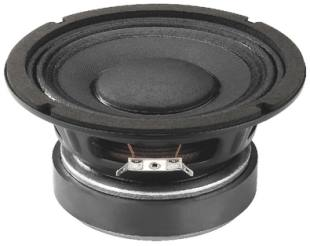recommended cabinet 1:
closed cabinet with 2,8 L volume
from 165/104 Hz (-3dB/-8dB)

recommended cabinet 2:
11,2 L volume bass reflex cabinet
with HP70 reflex tube, 8 cm long.
from 79/65 Hz (-3dB/-8dB).

SP-6/100PA       order no. mo-101670       EUR 48,05excl. VAT: € 40.38 / \$ 43.61
16 cm midrange driver with high quality cone.
• power handling (continuous/programme) = 100/200 W
• frequency range = 100-8000 Hz
• resonance frequency fs = 90 Hz
• impedance R = 8 Ohm
• sound pressure level SPL = 94 dB (2,83V; 1m)
• DC resistance Re = 6,7 Ohm
• force factor BL = 9,3 N/A
• voice coil inductance L = 0,5 mH
• effective piston radiating area Sd = 139 cm2
• effective mechanical mass incl. air load mms = 11,2 g
• equivalent volume of compliance Vas = 8 l
• total Q factor Qts = 0,44 (Qms=4,24, Qes=0,49)
• voice coil diameter = 40 mm
• mounting diameter d = 148 mm
• overall diameter d = 170 mm
• mounting depth (not countersunk) t = 79 mmrecommended cabinet 1:
closed cabinet with 2,8 L volume
from 137/86 Hz (-3dB/-8dB)

recommended cabinet 2:
11 L volume bass reflex cabinet
with HP70 reflex tube, 15 cm long.
from 66/54 Hz (-3dB/-8dB).

SP-6/150PA       order no. mo-103180       EUR 54,96excl. VAT: € 46.18 / \$ 49.88
16 cm midrange driver with high quality cone.
• power handling (continuous/programme) = 150/300 W
• frequency range = 80-6000 Hz
• resonance frequency fs = 73 Hz
• impedance R = 8 Ohm
• sound pressure level SPL = 93 dB (2,83V; 1m)
• DC resistance Re = 6,5 Ohm
• force factor BL = 9,9 N/A
• voice coil inductance L = 0,6 mH
• effective piston radiating area Sd = 139 cm2
• effective mechanical mass incl. air load mms = 15,2 g
• equivalent volume of compliance Vas = 8,5 l
• total Q factor Qts = 0,42 (Qms=5,31, Qes=0,46)
• voice coil diameter = 50 mm
• mounting diameter d = 148 mm
• overall diameter d = 170 mm
• mounting depth (not countersunk) t = 79 mmSP-165PA       order no. mo-103090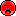- not available
16 cm midrange driver with high quality cone.
• power handling (continuous/programme) = 50/100 W
• frequency range = 30-5500 Hz
• resonance frequency fs = 100 Hz
• impedance R = 8 Ohm
• sound pressure level SPL = 90 dB (2,83V; 1m)
• mounting diameter d = 148 mm
• overall diameter d = 170 mm
• mounting depth (not countersunk) t = 80 mmrecommended cabinet 1:
closed cabinet with 4 L volume
from 165/104 Hz (-3dB/-8dB)

recommended cabinet 2:
16 L volume bass reflex cabinet
with HP100 reflex tube, 14 cm long.
from 80/65 Hz (-3dB/-8dB).

SP-8/150PA       order no. mo-101680       EUR 68,74excl. VAT: € 57.76 / \$ 62.39
20 cm midrange driver with high quality cone.
• power handling (continuous/programme) = 150/300 W
• frequency range = 90-8000 Hz
• resonance frequency fs = 88 Hz
• impedance R = 8 Ohm
• sound pressure level SPL = 96 dB (2,83V; 1m)
• DC resistance Re = 6,9 Ohm
• force factor BL = 12,6 N/A
• voice coil inductance L = 0,6 mH
• effective piston radiating area Sd = 219 cm2
• effective mechanical mass incl. air load mms = 20 g
• equivalent volume of compliance Vas = 12 l
• total Q factor Qts = 0,43 (Qms=4,12, Qes=0,48)
• voice coil diameter = 50 mm
• mounting diameter d = 185 mm
• overall diameter d = 210 mm
• mounting depth (not countersunk) t = 90 mmrecommended cabinet 1:
closed cabinet with 3,5 L volume
from 140/88 Hz (-3dB/-8dB)

recommended cabinet 2:
11 L volume bass reflex cabinet
with HP70 reflex tube, 14 cm long.
from 75/58 Hz (-3dB/-8dB).

SP-8/150PRO       order no. mo-100710       EUR 77,90excl. VAT: € 65.46 / \$ 70.70
20 cm bass driver with cellulose cone. The spider is rear ventilated for better cooling. The basket is made of die-cast.
• power handling (continuous/programme) = 150/300 W
• frequency range = 3-3000 Hz
• resonance frequency fs = 56 Hz
• impedance R = 8 Ohm
• sound pressure level SPL = 93 dB (2,83V; 1m)
• DC resistance Re = 7,2 Ohm
• force factor BL = 11,96 N/A
• voice coil inductance L = 2 mH
• effective piston radiating area Sd = 213 cm2
• effective mechanical mass incl. air load mms = 22,8 g
• equivalent volume of compliance Vas = 22 l
• total Q factor Qts = 0,32 (Qms=1,52, Qes=0,41)
• voice coil diameter = 50 mm
• mounting diameter d = 185 mm
• overall diameter d = 210 mm
• mounting depth (not countersunk) t = 100 mm
• mass m = 1,9 kg
PDF data sheetSP-200PA       order no. mo-102960- not available
20 cm midrange driver with high quality cone.
• power handling (continuous/programme) = 100/200 W
• frequency range = 30-4000 Hz
• resonance frequency fs = 55 Hz
• impedance R = 8 Ohm
• sound pressure level SPL = 91 dB (2,83V; 1m)
• mounting diameter d = 182 mm
• overall diameter d = 210 mm
• mounting depth (not countersunk) t = 90 mmrecommended cabinet 1:
closed cabinet with 4 L volume
from 170/108 Hz (-3dB/-8dB)

recommended cabinet 2:
11 L volume bass reflex cabinet
with HP100 reflex tube, 21 cm long.
from 97/74 Hz (-3dB/-8dB).

SP-10/150PA       order no. mo-102500       - not available (_)
24 cm midrange driver with high quality cone.
• power handling (continuous/programme) = 150/300 W
• frequency range = 60-5000 Hz
• resonance frequency fs = 60 Hz
• impedance R = 8 Ohm
• sound pressure level SPL = 98 dB (2,83V; 1m)
• DC resistance Re = 6,9 Ohm
• force factor BL = 18,2 N/A
• voice coil inductance L = 0,94 mH
• effective piston radiating area Sd = 350 cm2
• effective mechanical mass incl. air load mms = 38 g
• equivalent volume of compliance Vas = 34 l
• total Q factor Qts = 0,28 (Qms=5,36, Qes=0,3)
• voice coil diameter = 65 mm
• mounting diameter d = 228 mm
• overall diameter d = 258 mm
• mounting depth (not countersunk) t = 118 mmopen a bigger photo

SP-250PA       order no. mo-102970- not available
24 cm midrange driver with high quality cone.
• power handling (continuous/programme) = 125/250 W
• frequency range = 30-4000 Hz
• resonance frequency fs = 48 Hz
• impedance R = 8 Ohm
• sound pressure level SPL = 92 dB (2,83V; 1m)
• mounting diameter d = 232 mm
• overall diameter d = 255 mm
• mounting depth (not countersunk) t = 110 mmrecommended cabinet 1:
closed cabinet with 7,7 L volume
from 137/87 Hz (-3dB/-8dB)

recommended cabinet 2:
28 L volume bass reflex cabinet
with HP100 reflex tube, 10 cm long.
from 70/55 Hz (-3dB/-8dB).

SP-10/100PA       order no. mo-102490       - not available (_)
25 cm midrange driver with high quality cone.
• power handling (continuous/programme) = 100/200 W
• frequency range = 70-5000 Hz
• resonance frequency fs = 65 Hz
• impedance R = 8 Ohm
• sound pressure level SPL = 98 dB (2,83V; 1m)
• DC resistance Re = 6,9 Ohm
• force factor BL = 14,4 N/A
• voice coil inductance L = 0,75 mH
• effective piston radiating area Sd = 350 cm2
• effective mechanical mass incl. air load mms = 31 g
• equivalent volume of compliance Vas = 32 l
• total Q factor Qts = 0,38 (Qms=4,1, Qes=0,42)
• voice coil diameter = 50 mm
• mounting diameter d = 234 mm
• overall diameter d = 258 mm
• mounting depth (not countersunk) t = 106 mmrecommended cabinet 1:
closed cabinet with 5,5 L volume
from 146/92 Hz (-3dB/-8dB)

recommended cabinet 2:
16 L volume bass reflex cabinet
with HP100 reflex tube, 19 cm long.
from 81/62 Hz (-3dB/-8dB).

SP-10A/302PA       order no. mo-104920       EUR 150,00excl. VAT: € 126.05 / \$ 136.13
25 cm bass-midrange driver with high quality cone. The basket is made of die-cast. To avoid compression effects the driver possesses a pole piece hole.
• power handling (continuous/programme) = 300/600 W
• frequency range = 30-3500 Hz
• resonance frequency fs = 55 Hz
• impedance R = 8 Ohm
• sound pressure level SPL = 98 dB (2,83V; 1m)
• DC resistance Re = 6,4 Ohm
• force factor BL = 15,82 N/A
• voice coil inductance L = 0,65 mH
• effective piston radiating area Sd = 346 cm2
• effective mechanical mass incl. air load mms = 35 g
• equivalent volume of compliance Vas = 40 l
• total Q factor Qts = 0,3 (Qms=7,44, Qes=0,31)
• voice coil diameter = 66 mm
• mounting diameter d = 234 mm
• overall diameter d = 260 mm
• mounting depth (not countersunk) t = 112 mm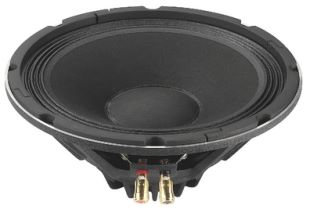SP-10A/250NEO       order no. mo-104600       - not available (_)
25 cm bass-midrange driver with high quality cone. The magnet system is based on Neodymium technology. The basket is made of die-cast.
• power handling (continuous/programme) = 250/500 W
• frequency range = 30-3000 Hz
• resonance frequency fs = 80 Hz
• impedance R = 8 Ohm
• sound pressure level SPL = 99 dB (2,83V; 1m)
• DC resistance Re = 5,7 Ohm
• force factor BL = 12,01 N/A
• voice coil inductance L = 0,3 mH
• effective piston radiating area Sd = 347 cm2
• effective mechanical mass incl. air load mms = 32 g
• equivalent volume of compliance Vas = 19 l
• total Q factor Qts = 0,63 (Qms=16,59, Qes=0,66)
• voice coil diameter = 50 mm
• mounting diameter d = 234 mm
• overall diameter d = 260 mm
• mounting depth (not countersunk) t = 110 mmrecommended cabinet 1:
closed cabinet with 12,8 L volume
from 115/73 Hz (-3dB/-8dB)

recommended cabinet 2:
49 L volume bass reflex cabinet
with HP100 reflex tube, 6 cm long.
from 56/46 Hz (-3dB/-8dB).

SPA-25PA       order no. mo-104030       - not available (_)
25 cm midrange driver with paper cone. The basket is made of die-cast.
• power handling (continuous/programme) = 125/250 W
• frequency range = 60-6000 Hz
• resonance frequency fs = 60 Hz
• impedance R = 8 Ohm
• sound pressure level SPL = 97 dB (2,83V; 1m)
• DC resistance Re = 5,7 Ohm
• force factor BL = 10,87 N/A
• voice coil inductance L = 0,5 mH
• effective piston radiating area Sd = 52 cm2
• effective mechanical mass incl. air load mms = 29 g
• equivalent volume of compliance Vas = 41 l
• total Q factor Qts = 0,42 (Qms=2,23, Qes=0,52)
• voice coil diameter = 50 mm
• mounting diameter d = 235 mm
• overall diameter d = 265 mm
• mounting depth (not countersunk) t = 99 mmrecommended cabinet 1:
closed cabinet with 27 L volume
from 95/60 Hz (-3dB/-8dB)

recommended cabinet 2:
96 L volume bass reflex cabinet
with 2x HP100 reflex tube, 9 cm long.
from 49/39 Hz (-3dB/-8dB).

SP-30/200PA       order no. mo-103350       EUR 116,80excl. VAT: € 98.15 / \$ 106.00
29 cm midrange driver with high quality cone.
• power handling (continuous/programme) = 200/400 W
• frequency range = 50-4500 Hz
• resonance frequency fs = 44 Hz
• impedance R = 8 Ohm
• sound pressure level SPL = 97 dB (2,83V; 1m)
• DC resistance Re = 6,5 Ohm
• force factor BL = 13,9 N/A
• voice coil inductance L = 0,9 mH
• effective piston radiating area Sd = 540 cm2
• effective mechanical mass incl. air load mms = 44 g
• equivalent volume of compliance Vas = 120 l
• total Q factor Qts = 0,37 (Qms=6,71, Qes=0,39)
• voice coil diameter = 51 mm
• mounting diameter d = 279 mm
• overall diameter d = 310 mm
• mounting depth (not countersunk) t = 132 mm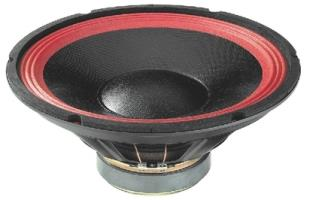SP-300PA       order no. mo-102980- not available
29 cm bass-midrange driver with high quality cone.
• power handling (continuous/programme) = 175/350 W
• frequency range = 30-4000 Hz
• resonance frequency fs = 40 Hz
• impedance R = 8 Ohm
• sound pressure level SPL = 93 dB (2,83V; 1m)
• mounting diameter d = 280 mm
• overall diameter d = 305 mm
• mounting depth (not countersunk) t = 130 mmSP-304PA       order no. mo-103950       EUR 104,42excl. VAT: € 87.75 / \$ 94.77
29 cm bass-midrange driver with high quality cone.
• power handling (continuous/programme) = 175/350 W
• frequency range = 30-4000 Hz
• resonance frequency fs = 40 Hz
• impedance R = 4 Ohm
• sound pressure level SPL = 93 dB (2,83V; 1m)
• mounting diameter d = 280 mm
• overall diameter d = 305 mm
• mounting depth (not countersunk) t = 130 mmrecommended cabinet 1:
closed cabinet with 13,2 L volume
from 121/76 Hz (-3dB/-8dB)

recommended cabinet 2:
42 L volume bass reflex cabinet
with 2x HP100 reflex tube, 17 cm long.
from 65/50 Hz (-3dB/-8dB).

SP-12A/302PA       order no. mo-104930       EUR 178,97excl. VAT: € 150.39 / \$ 162.43
30 cm bass-midrange driver with high quality cone. The basket is made of die-cast. To avoid compression effects the driver possesses a pole piece hole.
• power handling (continuous/programme) = 300/600 W
• frequency range = 30-4000 Hz
• resonance frequency fs = 50 Hz
• impedance R = 8 Ohm
• sound pressure level SPL = 99 dB (2,83V; 1m)
• DC resistance Re = 6,4 Ohm
• force factor BL = 16,98 N/A
• voice coil inductance L = 0,8 mH
• effective piston radiating area Sd = 530 cm2
• effective mechanical mass incl. air load mms = 50 g
• equivalent volume of compliance Vas = 77 l
• total Q factor Qts = 0,33 (Qms=7,87, Qes=0,34)
• voice coil diameter = 66 mm
• mounting diameter d = 284 mm
• overall diameter d = 313 mm
• mounting depth (not countersunk) t = 123 mm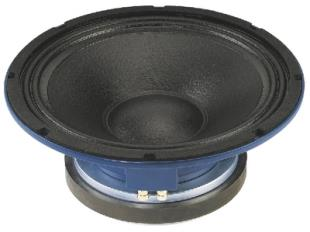recommended cabinet 1:
closed cabinet with 5,3 L volume
from 171/108 Hz (-3dB/-8dB)

recommended cabinet 2:
12,3 L volume bass reflex cabinet
with HP100 reflex tube, 18 cm long.
from 104/78 Hz (-3dB/-8dB).

SP-30A/250PA       order no. mo-103360       - not available (_)
30 cm bass-midrange driver with high quality cone.
• power handling (continuous/programme) = 250/1000 W
• frequency range = 30-4500 Hz
• resonance frequency fs = 52 Hz
• impedance R = 8 Ohm
• sound pressure level SPL = 100 dB (2,83V; 1m)
• DC resistance Re = 5,4 Ohm
• force factor BL = 20,3 N/A
• voice coil inductance L = 0,9 mH
• effective piston radiating area Sd = 530 cm2
• effective mechanical mass incl. air load mms = 60 g
• equivalent volume of compliance Vas = 62 l
• total Q factor Qts = 0,24 (Qms=5,55, Qes=0,25)
• voice coil diameter = 76 mm
• mounting diameter d = 283 mm
• overall diameter d = 318 mm
• mounting depth (not countersunk) t = 122 mmrecommended cabinet 1:
closed cabinet with 5,1 L volume
from 171/108 Hz (-3dB/-8dB)

recommended cabinet 2:
11,9 L volume bass reflex cabinet
with HP100 reflex tube, 19 cm long.
from 104/78 Hz (-3dB/-8dB).

SP-30A/300NEO       order no. mo-104310       - not available (_)
30 cm bass-midrange driver with high quality cone. The magnet system is based on Neodymium technology.
• power handling (continuous/programme) = 300/600 W
• frequency range = 30-3500 Hz
• resonance frequency fs = 52 Hz
• impedance R = 8 Ohm
• sound pressure level SPL = 100 dB (2,83V; 1m)
• DC resistance Re = 5,1 Ohm
• force factor BL = 20,1 N/A
• voice coil inductance L = 1 mH
• effective piston radiating area Sd = 530 cm2
• effective mechanical mass incl. air load mms = 60 g
• equivalent volume of compliance Vas = 60 l
• total Q factor Qts = 0,24 (Qms=5,36, Qes=0,25)
• voice coil diameter = 75 mm
• mounting diameter d = 280 mm
• overall diameter d = 314 mm
• mounting depth (not countersunk) t = 136 mmrecommended cabinet 1:
closed cabinet with 24 L volume
from 113/72 Hz (-3dB/-8dB)

recommended cabinet 2:
74 L volume bass reflex cabinet
with 2x HP100 reflex tube, 8 cm long.
from 62/48 Hz (-3dB/-8dB).

SP-38A/300NEO       order no. mo-104320       - not available (_)
37 cm bass-midrange driver with high quality cone. The magnet system is based on Neodymium technology.
• power handling (continuous/programme) = 300/600 W
• frequency range = 30-3500 Hz
• resonance frequency fs = 44 Hz
• impedance R = 8 Ohm
• sound pressure level SPL = 102 dB (2,83V; 1m)
• DC resistance Re = 5,2 Ohm
• force factor BL = 18,04 N/A
• voice coil inductance L = 0,9 mH
• effective piston radiating area Sd = 854 cm2
• effective mechanical mass incl. air load mms = 74 g
• equivalent volume of compliance Vas = 164 l
• total Q factor Qts = 0,31 (Qms=4,02, Qes=0,34)
• voice coil diameter = 75 mm
• mounting diameter d = 353 mm
• overall diameter d = 389 mm
• mounting depth (not countersunk) t = 165 mmrecommended cabinet 1:
closed cabinet with 13,9 L volume
from 128/81 Hz (-3dB/-8dB)

recommended cabinet 2:
41 L volume bass reflex cabinet
with 2x HP100 reflex tube, 16 cm long.
from 71/54 Hz (-3dB/-8dB).

SP-38A/300PA       order no. mo-103390       - not available (_)
38 cm bass-midrange driver with high quality cone.
• power handling (continuous/programme) = 300/1200 W
• frequency range = 30-4000 Hz
• resonance frequency fs = 48 Hz
• impedance R = 8 Ohm
• sound pressure level SPL = 102 dB (2,83V; 1m)
• DC resistance Re = 5,4 Ohm
• force factor BL = 24,1 N/A
• voice coil inductance L = 1,3 mH
• effective piston radiating area Sd = 880 cm2
• effective mechanical mass incl. air load mms = 100 g
• equivalent volume of compliance Vas = 101 l
• total Q factor Qts = 0,3 (Qms=7,45, Qes=0,31)
• voice coil diameter = 76 mm
• mounting diameter d = 360 mm
• overall diameter d = 391 mm
• mounting depth (not countersunk) t = 162 mmopen a bigger photo

recommended cabinet 1:
closed cabinet with 28 L volume
from 102/64 Hz (-3dB/-8dB)

recommended cabinet 2:
113 L volume bass reflex cabinet
with 2x HP100 reflex tube, 4 cm long.
from 48/40 Hz (-3dB/-8dB).

TRUVOX-1225       order no. mo-104990       - not available (_)
30 cm bass driver with cellulose cone.
• power handling (continuous/programme) = 250/500 W
• frequency range = 90-4000 Hz
• resonance frequency fs = 62 Hz
• impedance R = 8 Ohm
• sound pressure level SPL = 99 dB (2,83V; 1m)
• DC resistance Re = 5,3 Ohm
• force factor BL = 13,72 N/A
• voice coil inductance L = 0,8 mH
• effective piston radiating area Sd = 551 cm2
• effective mechanical mass incl. air load mms = 46 g
• equivalent volume of compliance Vas = 57 l
• total Q factor Qts = 0,48 (Qms=6,57, Qes=0,52)
• voice coil diameter = 63 mm
• mounting diameter d = 282 mm
• overall diameter d = 310 mm
• mounting depth (not countersunk) t = 130 mm
• mass m = 3,9 kgopen a bigger photo

recommended cabinet 1:
closed cabinet with 7,4 L volume
from 155/98 Hz (-3dB/-8dB)

recommended cabinet 2:
28 L volume bass reflex cabinet
with HP100 reflex tube, 6 cm long.
from 77/62 Hz (-3dB/-8dB).

TRUVOX-1020       order no. mo-104980       - not available (_)
24 cm midrange driver with cellulose cone.
• power handling (continuous/programme) = 150/300 W
• frequency range = 110-3500 Hz
• resonance frequency fs = 77 Hz
• impedance R = 8 Ohm
• sound pressure level SPL = 100 dB (2,83V; 1m)
• DC resistance Re = 5,5 Ohm
• force factor BL = 13,53 N/A
• voice coil inductance L = 0,7 mH
• effective piston radiating area Sd = 363 cm2
• effective mechanical mass incl. air load mms = 29 g
• equivalent volume of compliance Vas = 27 l
• total Q factor Qts = 0,39 (Qms=4,79, Qes=0,43)
• voice coil diameter = 50 mm
• mounting diameter d = 227 mm
• overall diameter d = 256 mm
• mounting depth (not countersunk) t = 108 mm
• mass m = 3,6 kgopen a bigger photo

TRUVOX-0818MR       order no. mo-104970       - not available (_)
19 cm midrange driver with cellulose cone.
• power handling (continuous/programme) = 100/200 W
• frequency range = 700-4500 Hz
• resonance frequency fs = 450 Hz
• impedance R = 8 Ohm
• sound pressure level SPL = 102 dB (2,83V; 1m)
• DC resistance Re = 6,7 Ohm
• voice coil inductance L = 0,7 mH
• effective piston radiating area Sd = 255 cm2
• voice coil diameter = 44 mm
• mounting diameter d = 181 mm
• overall diameter d = 208 mm
• mounting depth (not countersunk) t = 83 mm
• mass m = 1,85 kgopen a bigger photo

TRUVOX-0615MR       order no. mo-104960       - not available (_)
16 cm midrange driver with cellulose cone.
• power handling (continuous/programme) = 50/100 W
• frequency range = 800-5000 Hz
• resonance frequency fs = 520 Hz
• impedance R = 8 Ohm
• sound pressure level SPL = 98 dB (2,83V; 1m)
• DC resistance Re = 5,7 Ohm
• voice coil inductance L = 0,8 mH
• effective piston radiating area Sd = 169 cm2
• voice coil diameter = 38 mm
• mounting diameter d = 144 mm
• overall diameter d = 180 mm
• mounting depth (not countersunk) t = 73 mm
• mass m = 1,4 kgopen a bigger photo

TRUVOX-0510       order no. mo-104950       - not available (_)
13x13 cm midrange driver with cellulose cone.
• power handling (continuous/programme) = 35/75 W
• frequency range = 300-13000 Hz
• resonance frequency fs = 110 Hz
• impedance R = 8 Ohm
• sound pressure level SPL = 93 dB (2,83V; 1m)
• DC resistance Re = 6,2 Ohm
• force factor BL = 6,2 N/A
• voice coil inductance L = 0,33 mH
• effective piston radiating area Sd = 105 cm2
• effective mechanical mass incl. air load mms = 5,5 g
• equivalent volume of compliance Vas = 2,5 l
• total Q factor Qts = 0,51 (Qms=2,0, Qes=0,68)
• voice coil diameter = 25 mm
• mounting diameter d = 117 mm
• overall diameter d = 136x136 mm
• mounting depth (not countersunk) t = 68 mm
• mass m = 0,9 kgopen a bigger photo

recommended cabinet 1:
closed cabinet with 14 L volume
from 97/61 Hz (-3dB/-8dB)

recommended cabinet 2:
56 L volume bass reflex cabinet
with HP100 reflex tube, 10 cm long.
from 46/38 Hz (-3dB/-8dB).

SPA-10PA       order no. mo-105270       - not available (_)
25 cm bass-midrange driver with high quality cone. The basket is made of die-cast. To avoid compression effects the driver possesses a pole piece hole.
• power handling (continuous/programme) = 125/220 W
• frequency range = 80-3500 Hz
• resonance frequency fs = 54 Hz
• impedance R = 8 Ohm
• sound pressure level SPL = 95 dB (2,83V; 1m)
• DC resistance Re = 6,2 Ohm
• force factor BL = 12,49 N/A
• voice coil inductance L = 0,9 mH
• effective piston radiating area Sd = 346 cm2
• effective mechanical mass incl. air load mms = 39 g
• equivalent volume of compliance Vas = 37 l
• total Q factor Qts = 0,45 (Qms=2,79, Qes=0,53)
• voice coil diameter = 50 mm
• mounting diameter d = 232 mm
• overall diameter d = 262 mm
• mounting depth (not countersunk) t = 120 mm
• mass m = 3,4 kgopen a bigger photo

recommended cabinet 1:
closed cabinet with 4,3 L volume
from 129/81 Hz (-3dB/-8dB)

recommended cabinet 2:
17 L volume bass reflex cabinet
with HP100 reflex tube, 23 cm long.
from 61/51 Hz (-3dB/-8dB).

SPA-8PA       order no. mo-105260       EUR 58,23excl. VAT: € 48.93 / \$ 52.85
20 cm bass-midrange driver with high quality cone. The basket is made of die-cast. To avoid compression effects the driver possesses a pole piece hole.
• power handling (continuous/programme) = 80/150 W
• frequency range = 125-4000 Hz
• resonance frequency fs = 75 Hz
• impedance R = 8 Ohm
• sound pressure level SPL = 94 dB (2,83V; 1m)
• DC resistance Re = 6,2 Ohm
• force factor BL = 12,26 N/A
• voice coil inductance L = 0,8 mH
• effective piston radiating area Sd = 213 cm2
• effective mechanical mass incl. air load mms = 27 g
• equivalent volume of compliance Vas = 10 l
• total Q factor Qts = 0,46 (Qms=3,13, Qes=0,54)
• voice coil diameter = 50 mm
• mounting diameter d = 184 mm
• overall diameter d = 210 mm
• mounting depth (not countersunk) t = 105 mm
• mass m = 3,1 kgopen a bigger photo

SP-165GI       order no. mo-102270       - not available (_)
15 cm midrange driver with high quality cone.
• power handling (continuous/programme) = 60/30 W
• frequency range = 135-8000 Hz
• resonance frequency fs = 90 Hz
• impedance R = 8 Ohm
• sound pressure level SPL = 94 dB (2,83V; 1m)
• mounting diameter d = 146 mm
• overall diameter d = 165 mm
• mounting depth (not countersunk) t = 75 mm
• mass m = 1,2 kg
 Tweeter Mids Fullrange Woofer no longer available PA Mids Woofer Fullrange no longer available Car Woofer no longer available
The cabinet volume calculations are executed considering an external resistance of 0.3 Ohm. We are using, if no other data is known or available, the information supplied by the manufacturer.

Please note: the frequency response doesn't say much about the cut-off frequency inside the cabinet! Therefore, we mostly give you 2 examples with dimensioning considerations and an indication, how low the loudspeaker will reproduce.

Home